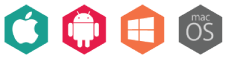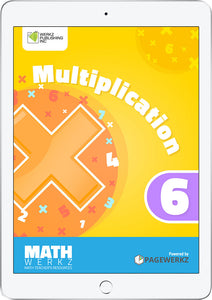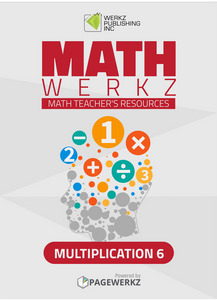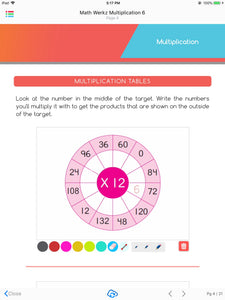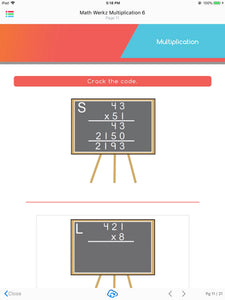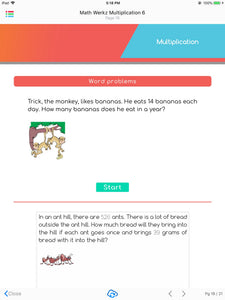# Math Werkz Multiplication 6

Regular price \$2.00 Sale

Product Description:

Math Werkz Multiplication 6 is a fun, interactive courseware that provides students a go-to workbook on Multiplication.

Through this ebook, kids can now understand how to multiply numbers through the use of multiplication chart, arrays and patterns, with the addition of essential worksheets on correspondence and word problems. Among the activities included are answering multiplication tables, solving word problems, calculating and multiplying numbers, and understanding multiplication tricks for easy calculation. Teachers can also use this as additional exercises or classroom-based activities for their students to work on.

Math Werkz Multiplication 6 is one of six Math Werkz Multiplication interactive eBooks.

Math Werkz Series is a Primary Mathematics Teacher Resource consisting of 90+ activity-based worksheets. These worksheets have teacher-enabled tools that can be used to teach and engage students using any available devices like tablets, iPads, smartphones, or classroom devices like whiteboards, SMART Boards or PCs.

Overall, there is a total of 98 Math Werkz interactive eBooks available with topics that range from Factors & Multiples, Fraction, Money, Time, Division, Geometry, Measurement, Graphs and Charts, Multiplication, Percentage, Decimal, Area and Perimeter. Assessment Book, Addition, Subtraction and Number System.

Author: Werkz Publishing Inc.

Number of pages: 21

Available platforms: